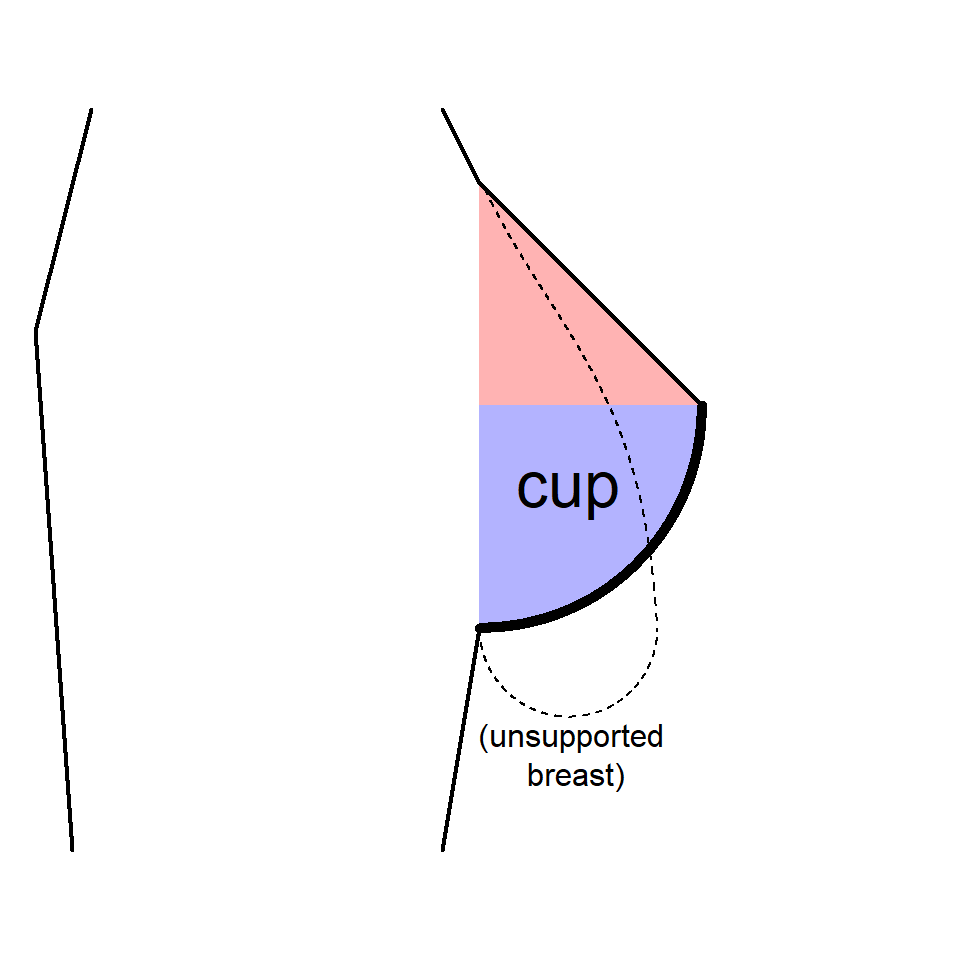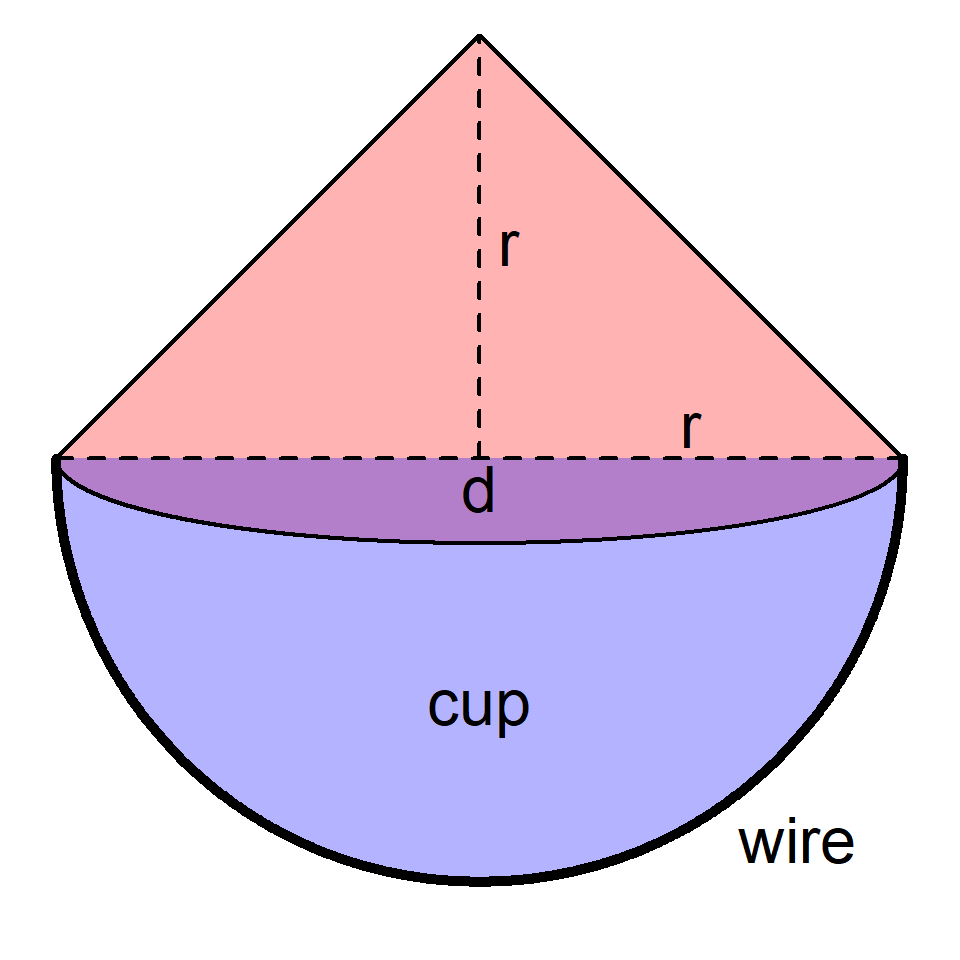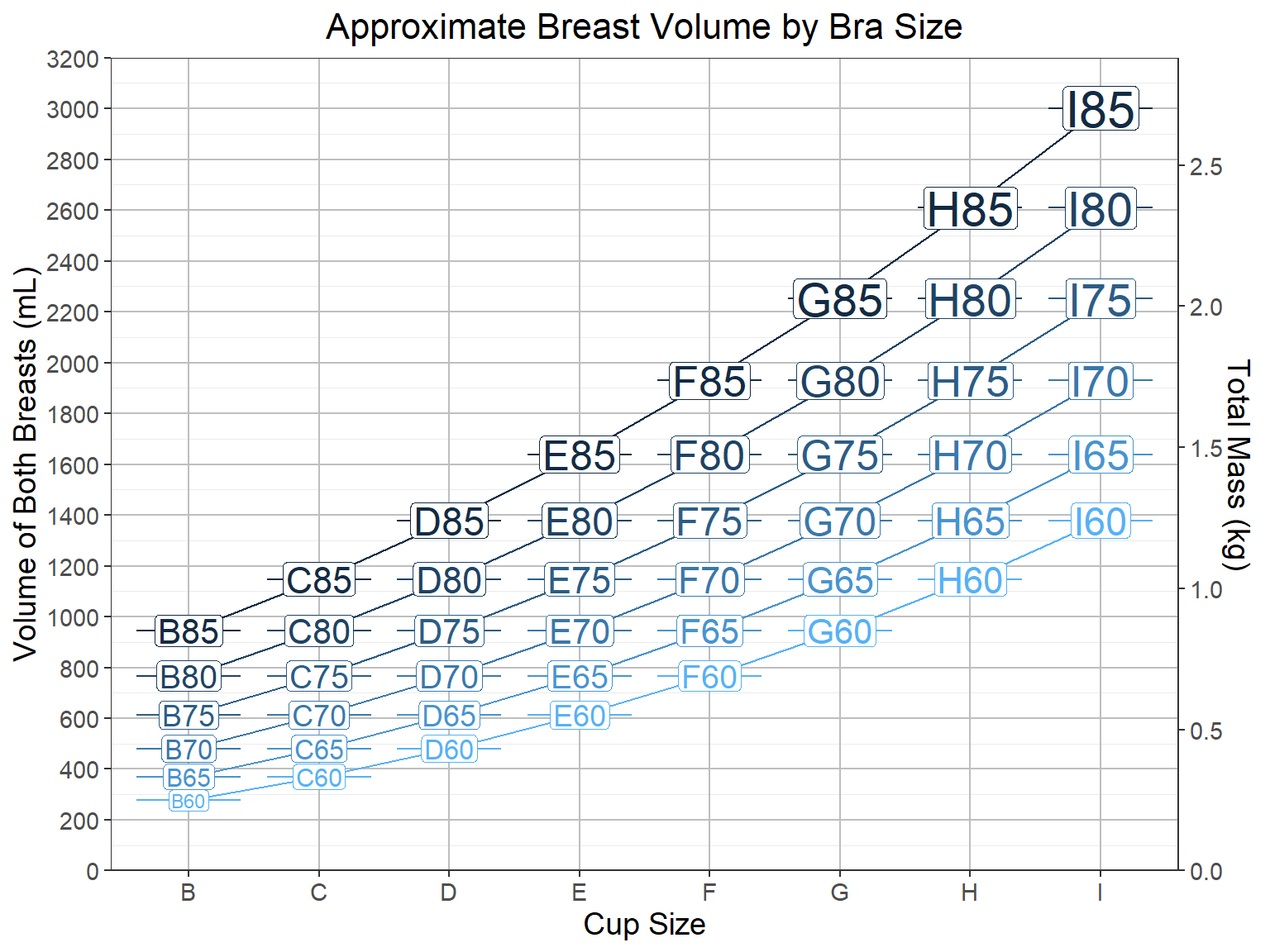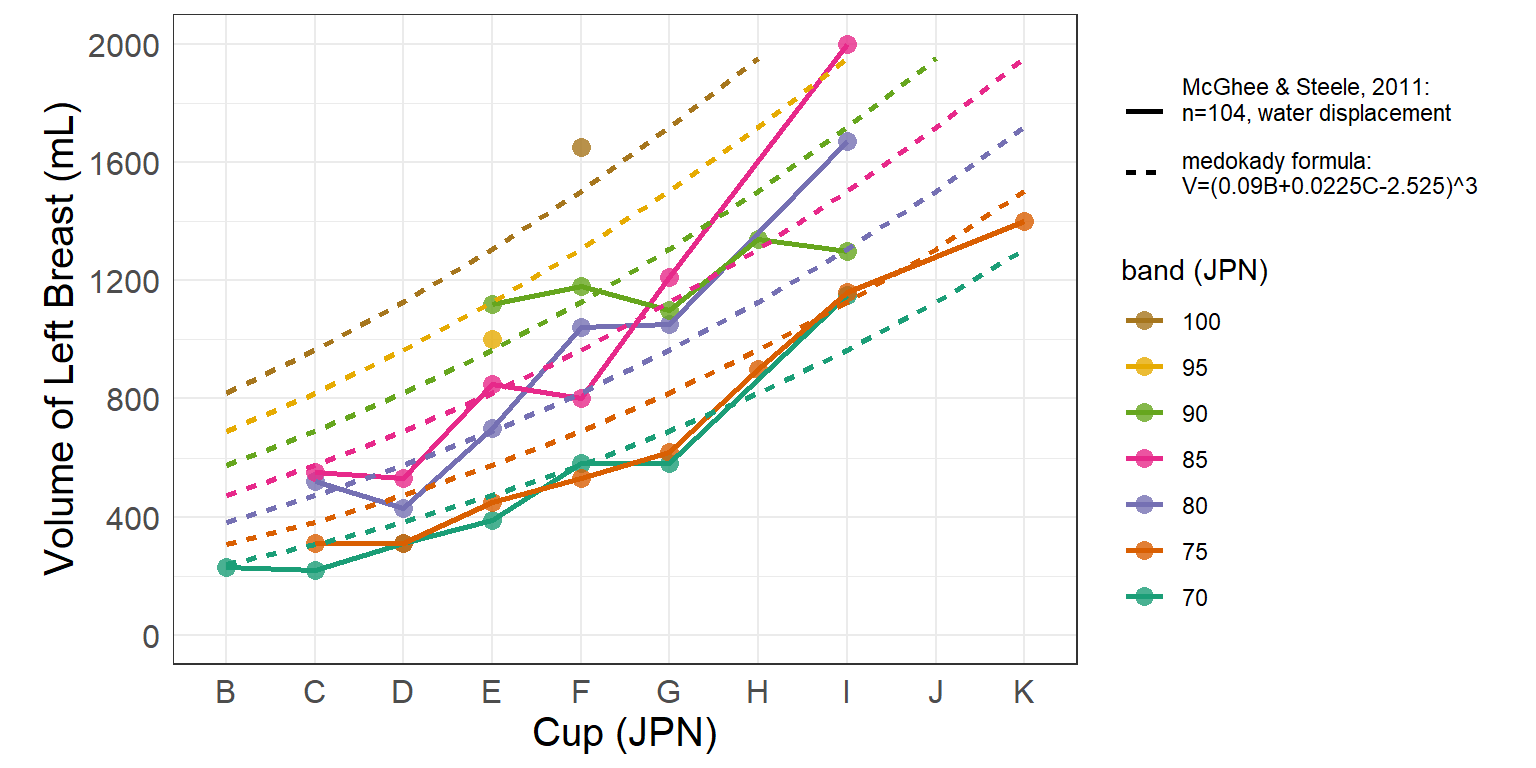# 🍒 Introduction

This document details the resources and steps used to generate an approximation of breast volume given bust and cup size. Ultimately, they yield easy to use formulas for calculating volume and mass. Perfect calculation, or even robust approximation, is practically impossible, but this should satisfy the desire to put numbers to visuals and compare volumes across wide differences in cup or bust size. Areas for improvement are also detailed.

# 🍊 Background: How Bras Work

At its core, a bra is a garment which supports weight. The straps lift breasts, distributing their weight across a woman’s shoulders and away from her chest. The dimensions of those straps are tailored to the weight and volume of the breasts they support. Thus it stands to reason that using those dimensions, one could estimate the volume of the breasts themselves.

## 📏 Measurements

All bra sizing methods rely on measurements, most notably bust and underbust.1 In short, bust is the circumference of the torso at the fullest part of the breasts. Underbust is the circumference of the torso right underneath where the breasts hang (not the waist) . Ideally, these measurements are made with the breasts in a supported position, as if they are already being suspended in a bra. Common advice is to measure while wearing one’s most comfortable bra .

## 👚 Bra Sizing

There are dozens of bra sizing systems around the world. All sizes in this document follow Japanese sizing, due to its mathematical simplicity. It can be described in five points :

• Bust size is the bust measurement in cm to the nearest 2.5 cm.
• Underbust size (a.k.a. band size) is the underbust measurement in cm to the nearest 5 cm.
• Cup size is the difference of the bust and underbust sizes.
• A cup size of 10 cm corresponds to an “A” cup, and every 2.5 cm increase marks the next letter cup.
• Finally, Bra size is expressed as the cup letter followed by the band size, e.g. B75.

As an example, consider a woman whose bust measurement is 96 cm and underbust measurement is 74 cm. Her bust size would be rounded down to 95 cm and her underbust would be rounded up to 75 cm, for a cup size of 20 cm. Being 4 steps of 2.5 cm above the 10 cm for A cup, 20 cm corresponds to E cup. Therefore the final size is E75.2

All bra sizing systems are variations on this theme, the major differences being units of measure and the progression scheme of the letters. Tables for converting between different sizing systems are available at sizeguide.net . Nevertheless, no matter the sizing system the fundamental mechanics of a bra and its purpose remain the same.

## 👯 Sister Sizing

Sister sizing is a principle whereby different bra sizes correspond to equal volumes. This is due to the fact that the breast shape changes with torso size, which affects total bust. In short, you can go up one cup and down one band or vice versa, without affecting volume .3 For example, given a bra size C70:

• The bra with one larger cup and one smaller band, D65, has the same volume.
• The bra with one smaller cup and one larger band, B75, has the same volume.

Sister size bras share volumes despite being otherwise different shapes because one crucial component of the bra is shared between them: the underwire. Underwires are U-shaped rigid bands sewn into the bottom of each cup which form the cup’s shape. Sister sizes share the same underwire length; therefore, the length of a C70 underwire is the same as that of a D65 or B75. Underwires are not usually expressed in raw lengths but “sizes,” calibrated to the American B (Japanese C) cup; therefore, a C70 has an “underwire size” of 70, as does D65 and B75 .

# 🍋 Calculations

Coming up with a formula will require two basic steps:

• Model breasts using geometric shapes
• Rewrite the formula for those shapes in terms of bust and cup size

## 📐 Geometry

While the natural breast shape is not easily defined by any combination of simple geometric shapes, the shape of one supported by a bra does allow for a robust approximation, which will form the basis of the formula. The shape of a breast in a bra can be divided into a top and bottom half; the former being a half-cone and the latter a quarter-sphere . The height and radius of the half-cone as well as the radius of the quarter-sphere will be designated as $$r$$ and the diameters as $$d$$:Breast in profile (left) and head-on (right). Red, half-cone; blue, quarter sphere.

Given that the formula for the volume of a cone is $$\frac{1}{3}\pi{r}^2{h}$$ (in this case $$h=r$$) and the formula for the volume of a sphere is $$\frac{4}{3}\pi{r}^3$$, a formula for the volume of two breasts can be written in terms of $$r$$ and simplified: \begin{aligned} V&=2\cdot\left[\frac{1}{2}\left(\frac{1}{3}\pi{r}^2{h}\right)^{cone}+\frac{1}{4}\left(\frac{4}{3}\pi{r}^3\right)^{sphere}\right] \\ &= 2\cdot\left[\frac{1}{6}\pi{r}^3+\frac{1}{3}\pi{r}^3\right] \\ &= \pi{r}^3 \end{aligned}

Surprisingly elegant.

## 💡 Strategy

The formula $$V=\pi{r}^3$$ must be rewritten in terms of the desired starting variables, bust and cup.

In order to calculate radius from bust and cup, it is necessary to:

1. Convert cup size in letters to cup size in cm
2. Calculate underbust from cup and bust
3. Calculate wire size from underbust and cup
4. Finally, measure the wire radius from wire size

To make these calculations more understandable they are worked through with this small dataset of 4 hypothetical women:

The lucky contestants.
bust (cm) cup
90 C
81 E
87 D
101 I

### ✖ Cup Size

First, cup letters must be converted to cup cm. This simply follows the scheme for Japanese sizing detailed in the previous section. Given the rules, a formula can be written to calculate the cm given the number of the cup letter in the alphabet (e.g., “A” is 1, “B” is 2, etc.): $cup_{cm} = 2.5\cdot{cup^{JPN}_{letter\#}}+7.5$

Which looks like this:

Japanese cup sizes and their bust-underbust difference.
cup cup (cm)
A 10
B 12.5
C 15
D 17.5
E 20
F 22.5
G 25
H 27.5
I 30
J 32.5

### ➕ Underbust and Bra Size

Now the cup letters in the sample data can be converted to cm (rounded to the nearest 2.5 cm), subtracted from the bust, and rounded to the nearest 5 cm in order to calculate the underbust: $underbust^{unrounded}_{cm} = bust_{cm}-cup_{cm}$

Combining the cup letter and underbust will yield the bra size. Using the sample data:

The newly calculated bra size is in the last column.
bust (cm) cup cup (cm) underbust (cm) bra size
90 C 15.0 75 C75
81 E 20.0 60 E60
87 D 17.5 70 D70
101 I 30.0 70 I70

### ➖ Wire Size

Now, wire size is calculated from the cup cm and underbust cm. The basic principle as stated in the previous section on sister sizing is that the wire of a C cup is equal to her own band size and that sister sizes share wire sizes. A formula to do so is as follows: $wire\ size = underbust_{cm}+2\cdot(cup_{cm}-15)$

Applying it to the sample data:

Notice how the two sister sizes share a wire size.
bust (cm) cup cup (cm) underbust (cm) bra size wire size
90 C 15.0 75 C75 75
81 E 20.0 60 E60 70
87 D 17.5 70 D70 75
101 I 30.0 70 I70 100

OK, so the formula uses wire sizes, but how does that relate to their actual physical dimensions? A primary source for wire dimensions was used as a reference (full details may be found in the methods) . The measurements, converted to Japanese sizing, are as follows:

Lengths are direct from source, diameter is self-measured.
wire wire length (cm) wire diameter (cm) wire radius (cm)
55 15.3 8.9 4.45
60 16.8 9.8 4.9
65 18.3 10.7 5.35
70 19.8 11.6 5.8
75 21.3 12.5 6.25
80 22.8 13.4 6.7
85 24.3 14.3 7.15
90 25.8 15.2 7.6
95 27.3 16.1 8.05
100 28.8 17 8.5

A quick calculation tells us that the formula for wire radius $$(r)$$ given wire size $$(s)$$ is $$r=0.09\cdot{s}-0.5$$.

Applying to the sample data:

Almost there!
bust (cm) cup cup (cm) underbust (cm) bra size wire size wire radius (cm)
90 C 15.0 75 C75 75 6.25
81 E 20.0 60 E60 70 5.80
87 D 17.5 70 D70 75 6.25
101 I 30.0 70 I70 100 8.50

### 🧮 Putting it Together

Now that formulas have been constructed from bust and cup size until radius, all that is needed is to combine all those formulas together with the original $$V=\pi{r}^3$$. Replacing each piece step by step in reverse order from how they were added: \begin{aligned} V_{mL} &= \pi{r}^3 \\ &= \pi\cdot(0.09\cdot{wire\ size}-0.5)^3 \\ &= \pi\cdot(0.09\cdot[underbust_{cm} + 2\cdot(cup_{cm} - 15)]-0.5)^3 \\ &= \pi\cdot(0.09\cdot[(bust_{cm} - cup_{cm}) + 2\cdot(cup_{cm} - 15)]-0.5)^3 \\ &= \pi\cdot(0.09\cdot[(bust_{cm} - [2.5\cdot cup^{JPN}_{letter\#} + 7.5]) + 2\cdot([2.5\cdot cup^{JPN}_{letter\#} + 7.5] - 15)]-0.5)^3 \\ \end{aligned}

Give it a health dose of simplification, and the result is the final formula:4 $V_{mL} = \pi\cdot(0.09\cdot{bust_{cm}}+0.225\cdot{cup^{JPN}_{letter\#}}-2.525)^3$

The formula can now be tested with the sample data to find total volume. The result will in be units of cm3, a.k.a. milliliters (mL):

As expected, the sister size pair have similar volumes.
bust (cm) cup cup (cm) underbust (cm) bra size wire size wire radius (cm) volume (mL)
90 C 15.0 75 C75 75 6.25 767
81 E 20.0 60 E60 70 5.80 642
87 D 17.5 70 D70 75 6.25 751
101 I 30.0 70 I70 100 8.50 1991

Below is a visualization of how this formula scales with bra size:This plot highlights the profound effect of band size on total volume.

### 📝 Sidenote: Mass

For those interested in the mass of the breasts, the only extra piece of requisite information is the average density of breast tissue, which is roughly 0.9 g/cm3 . Therefore, based on the derived volume the formula for mass in kilograms would be: \begin{aligned} m_{kg} &= \frac{V_{mL}}{1000}\cdot0.9 \\ &= 0.0009\cdot{V}_{mL} \end{aligned}

# 🍑 Validation

So the formula makes sense and looks like it works, but how well does it really? It shall be put to the test by comparison to primary research data published in 2011 by McGhee and Steele . They measured the breast volumes of 104 women by water displacement and compared them to those women’s professionally-fitted bra sizes. Should the formula be accurate, then inputting the bra sizes of these women should yield volumes close to their actual values.

## 👀 Visual Comparison

Figure 2 of their paper is reproduced below, showing the variation in breast size with band and cup size (converted to the Japanese system). Overlaid on that graph with dotted lines are the corresponding values as obtained by the formula:Comparison of this formula to data collected by McGhee and Steele, 2011.

It must be said that there are of several obvious limitations to this validation strategy. While the sample size (n = 104) and range (200-2000 mL) are respectable, the paper does not give us the information to precisely determine how many breasts fell into each bin, so whether a point is representing one woman or ten is unknowable. Bra sizes at the extreme upper end probably had smaller sample sizes. Another, more significant limitation is the understanding that, as the paper confirms, bra size is not a perfect predictor of volume. A range of volumes correspond to one bra size, and there are times when larger cup size or band size mysteriously results in a lower volume (e.g.  E85 to F95, I85 and I90). Therefore, the current goal is only to see whether the data roughly fits. Most points are fairly close to the formula curve, and when they aren’t they seem to bee too high or too low in equal proportion.

## 📈 Correlation and Error

Between the real measured volumes and formula-predicted volumes, the r2 value is 0.86, the mean bias error is -21, the mean absolute error is 135, and the mean absolute percentage error is 18%. In other words, the formula explains 86% of the variability in volume seen in the sample, the formula has an underestimation bias of 21 mL, and the formula is off by an average of 135 mL, or 18%, of the correct volume. Given the level of variance in the source data, these metrics were found to be adequate, although they leave ample room for improvement. It is also unknown how the formula would perform for a different or broader sample.

# 🍏 Closing Remarks

## 🤏 Limitations

There are several:

1. The formula does not approximate breast volume as much as it approximates breast-in-a-bra volume. Ideally, a model for breast volume would follow the scheme laid out by Kayar et al., in which the three dimensions of the naked breast tissue were measured and used in order to calculate volume, independent of whatever bra supports them on a day to day basis . Unfortunately, such metrics do not exist for the vast majority of women, nor do women know them, nor do they have any presence in popular consciousness like bust and cup size do.
2. Sister sizing is only a tool for women to find a bra that fits them better, and does not really work infinitely as suggested. Depending on breast shape, the degree to which they fill the bra will change. This could be remedied by devising some scaling factor dependent on cup or bust size, but such investigations are beyond the scope of this document.
3. The contribution of wire measurements to bust is probably overly simplified. At the most basic level, the diameters could probably stand to be measured more precisely. Also, the fact that the diameter is simply designated as the tip-to-tip distance for each size, as opposed to a proportion based on size, is a simplification.
4. The source of wire measurements is arbitrary. These measurements will vary by manufacturer.
5. There is a lack of data relating breast volume to bra size. The more data there is, the more the formula can be tested and fine-tuned. Nevertheless…
6. The variety of breast shapes precludes a highly-accurate estimation of volume based on any measurements derived from bras. In the words of McGhee and Steele, “a range of breast volumes was found to correspond to the same bra size” . There will be an upper limit on the accuracy of any simple formula like the one established here.

## 🔚 Conclusion

Bra size does not define volume, and breasts come in varied and non-mathematical shapes. Breasts are not like fluids, which fill the container they are given; therefore, trying to model their size based on the article of clothing that holds them is inherently ridiculous. The purpose of these calculations and formulas should only be to educate, entertain, and satiate curiosity.

Then again, maybe it’s the fact that they elude explanation which makes breasts beautiful.

# 🍈 Acknowledgments and Contact

I’d like to thank all of the sources I cited for giving me the tools I needed to attempt this task, the Bust Ranking Discord server for the inspiration to do it, and a certain girl🌸 whom I consulted for far too long without whom this would not be possible.

I am sincerely interested in improving upon the stated and unstated limitations of this approach and welcome any feedback or input. Feel free to hit me up @medokady#5874 on Discord.

# 📊 Appendix A: Formulas

## 🥛 Volume

For the volume of both breasts in mL, given bust in cm and Japanese cup size: $V_{mL} = \pi\cdot(0.09\cdot{bust_{cm}}+0.225\cdot{cup^{JPN}_{letter\#}}-2.525)^3$

For the volume of both breasts in mL, given Japanese bra size (band in cm and Japanese cup size): $V_{mL} = \pi\cdot(0.09\cdot{band_{cm}}+0.45\cdot{cup^{JPN}_{letter\#}}-1.85)^3$

## 🧱 Mass

For breast mass in kg, given breast volume in mL:

$m_{kg} = 0.0009\cdot{V}_{mL}$

# 🔧 Appendix B: Materials and Methods

Aside from the universally understood principles detailed in the background, several more specific (and more subjective) sources were used and are listed in elaborated detail below:

## ☕ Underwires

Printable underwire charts in American sizes, detailing their dimensions, can be found at The BraMakery . For this analysis the R1 regular day underwires were used. These enabled incorporation of real physical underwires measurements into the formulas. In order to convert these for use with Japanese sizes, all listed cups were increased by one and all band sizes converted to raw cm. Due to the fact that wires are not perfect semicircles, the diameters were measured manually instead of being calculated from the provided lengths.

## 👙 Breast Specs

### 🔊 Volume

To date, the most comprehensive study with the explicit aim of comparing bra size to volume is a 2011 article by McGhee and Steele . In their own words, “This is the first study to publish normative breast volume data, and the corresponding correctly fitted bra sizes, for a large sample of women.” This data served to test the formula against real measured data, so that it was not just theoretical. Australian sizes were converted to Japanese using the previously linked conversion chart. The data was extracted using WebPlotDigitizer .

### 🍮 Shape

Figure 1 of a 2006 article by McGhee and Steele provides an approximation of the geometric shape of breasts when contained in a bra, which is adapted in part of Figure 1 of this document .

### ⚖ Density

A 2011 article by Kayar et al. provides precise measurements of breast density for both normal and abnormal breasts . The value for breast density used (0.9 g/cm3) is rounded from the value for normal breasts.

## 👾 Code

Document compilation and data analysis were performed in R v4.1.0 using the packages here v1.0.1, tidyverse v1.3.1, knitr v1.33, and rmarkdown v2.10 .

1. For all things bra, a great first resource is the Wikipedia article on bra size .↩︎

2. Not E95! In almost every bra sizing system, the number corresponds to the band size, not the bust size.↩︎

3. For an understanding of exactly why this works (in theory), recall that band sizes increment by 5 cm and bust sizes by 2.5 cm. With each increase in band size, half of those cm go to lengthening the back, or “wings,” of the bra, while the other 2.5 cm go to increasing the bust and do so by one size. Therefore, if you drop the cup size by one in return, you can maintain breast volume.↩︎

4. This formula does not take into account rounding of the bust or band size, which occurs in real life for bra-fitting purposes. Practically, most women only know their measurements to the nearest bra size.↩︎